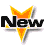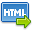Journal :   International Journal of Technology

Volume No. :   4

Issue No. :  1

Year :  2014

Pages :   134-139

ISSN Print :  2231-3907

ISSN Online :  2231-3915Registration

## Fuzzy Mixture Inventory Model Involving Fuzzy Lead Time Demand and Fuzzy Total Demand

 Address: S. Kumar*, B. Mukherjee Department of Applied Mathematics, Indian School of Mines, Dhanbad-826004, India .*Corresponding Author
DOI No:

ABSTRACT:
This paper is Fuzzy mixture inventory model involving fuzzy lead time demand and fuzzy total demand. The model is inventory model system ,Expected annual total cost in crisp sense calculated but lead time crashing cost ,holding cost ,stock out cost ,setup cost is assume as fuzzy random variable and total expected annual cost in fuzzy sense also calculated . Expected annual total cost is represented by Trapezoidal fuzzy number. We have used the Graded mean integration of defuzzification. Numerical example has been shown to compare the both result in crisp and fuzzy sense.
KEYWORDS:
Lead Time Crashing Cost; Demand; Trapezoidal Fuzzy Number; Expectated Annual Total Cost.
Cite:
S. Kumar*, B. Mukherjee. Fuzzy Mixture Inventory Model Involving Fuzzy Lead Time Demand and Fuzzy Total Demand. Int. J. Tech. 4(1): Jan.-June. 2014; Page 134-139[View HTML][View PDF]

Visitor's No. :   125042